### Bayesian Analysis of a Linear Regression Model

Neter et al. (1996) describe a study of 54 patients undergoing a certain kind of liver operation in a surgical unit. The data set Surg contains survival time and certain covariates for each patient. Observations for the first 20 patients in the data set Surg are shown in Figure 40.7.

Figure 40.7: Surgical Unit Data

Obs x1 x2 x3 x4 y logy Logx1
1 6.7 62 81 2.59 200 2.3010 1.90211
2 5.1 59 66 1.70 101 2.0043 1.62924
3 7.4 57 83 2.16 204 2.3096 2.00148
4 6.5 73 41 2.01 101 2.0043 1.87180
5 7.8 65 115 4.30 509 2.7067 2.05412
6 5.8 38 72 1.42 80 1.9031 1.75786
7 5.7 46 63 1.91 80 1.9031 1.74047
8 3.7 68 81 2.57 127 2.1038 1.30833
9 6.0 67 93 2.50 202 2.3054 1.79176
10 3.7 76 94 2.40 203 2.3075 1.30833
11 6.3 84 83 4.13 329 2.5172 1.84055
12 6.7 51 43 1.86 65 1.8129 1.90211
13 5.8 96 114 3.95 830 2.9191 1.75786
14 5.8 83 88 3.95 330 2.5185 1.75786
15 7.7 62 67 3.40 168 2.2253 2.04122
16 7.4 74 68 2.40 217 2.3365 2.00148
17 6.0 85 28 2.98 87 1.9395 1.79176
18 3.7 51 41 1.55 34 1.5315 1.30833
19 7.3 68 74 3.56 215 2.3324 1.98787
20 5.6 57 87 3.02 172 2.2355 1.72277

Consider the model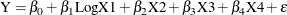where Y is the survival time, LogX1 is log(blood-clotting score), X2 is a prognostic index, X3 is an enzyme function test score, X4 is a liver function test score, andis an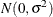error term.

A question of scientific interest is whether blood clotting score has a positive effect on survival time. Using PROC GENMOD, you can obtain a maximum likelihood estimate of the coefficient and construct a null point hypothesis to test whether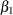is equal to 0. However, if you are interested in finding the probability that the coefficient is positive, Bayesian analysis offers a convenient alternative. You can use Bayesian analysis to directly estimate the conditional probability,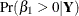, using the posterior distribution samples, which are produced as part of the output by PROC GENMOD.

The example that follows shows how to use PROC GENMOD to carry out a Bayesian analysis of the linear model with a normal error term. The SEED= option is specified to maintain reproducibility; no other options are specified in the BAYES statement. By default, a uniform prior distribution is assumed on the regression coefficients. The uniform prior is a flat prior on the real line with a distribution that reflects ignorance of the location of the parameter, placing equal likelihood on all possible values the regression coefficient can take. Using the uniform prior in the following example, you would expect the Bayesian estimates to resemble the classical results of maximizing the likelihood. If you can elicit an informative prior distribution for the regression coefficients, you should use the COEFFPRIOR= option to specify it. A default noninformative gamma prior is used for the scale parameter.

You should make sure that the posterior distribution samples have achieved convergence before using them for Bayesian inference. PROC GENMOD produces three convergence diagnostics by default. If ODS Graphics is enabled as specified in the following SAS statements, diagnostic plots are also displayed. See the section Assessing Markov Chain Convergence for more information about convergence diagnostics and their interpretation.

Summary statistics of the posterior distribution samples are produced by default. However, these statistics might not be sufficient for carrying out your Bayesian inference, and further processing of the posterior samples might be necessary. The following SAS statements request the Bayesian analysis, and the OUTPOST= option saves the samples in the SAS data set PostSurg for further processing:


proc genmod data=Surg;
model y = Logx1 X2 X3 X4 / dist=normal;
bayes seed=1 OutPost=PostSurg;
run;



The results of this analysis are shown in the following figures.

The Model Information table in Figure 40.8 summarizes information about the model you fit and the size of the simulation.

Figure 40.8: Model Information

The GENMOD Procedure

Bayesian Analysis

Model Information
Data Set WORK.SURG
Burn-In Size 2000
MC Sample Size 10000
Thinning 1
Sampling Algorithm Conjugate
Distribution Normal
Dependent Variable y Survival Time

The Analysis of Maximum Likelihood Parameter Estimates table in Figure 40.9 summarizes maximum likelihood estimates of the model parameters.

Figure 40.9: Maximum Likelihood Parameter Estimates

Analysis Of Maximum Likelihood Parameter Estimates
Parameter DF Estimate Standard Error Wald 95% Confidence Limits
Intercept 1 -730.559 85.4333 -898.005 -563.112
Logx1 1 171.8758 38.2250 96.9561 246.7954
x2 1 4.3019 0.5566 3.2109 5.3929
x3 1 4.0309 0.4996 3.0517 5.0100
x4 1 18.1377 12.0721 -5.5232 41.7986
Scale 1 59.8591 5.7599 49.5705 72.2832

 Note: The scale parameter was estimated by maximum likelihood.

Since no prior distributions for the regression coefficients were specified, the default noninformative uniform distributions shown in the Uniform Prior for Regression Coefficients table in Figure 40.10 are used. Noninformative priors are appropriate if you have no prior knowledge of the likely range of values of the parameters, and if you want to make probability statements about the parameters or functions of the parameters. See, for example, Ibrahim, Chen, and Sinha (2001) for more information about choosing prior distributions.

Figure 40.10: Regression Coefficient Priors

The GENMOD Procedure

Bayesian Analysis

Uniform Prior for Regression
Coefficients
Parameter Prior
Intercept Constant
Logx1 Constant
x2 Constant
x3 Constant
x4 Constant

The default noninformative improper prior distribution for the normal dispersion parameter is shown in the Independent Prior Distributions for Model Parameters table in Figure 40.11.

Figure 40.11: Scale Parameter Prior

Independent Prior Distributions
for Model Parameters
Parameter Prior Distribution
Dispersion Improper

By default, the maximum likelihood estimates of the regression parameters are used as the starting values for the simulation when noninformative prior distributions are used. These are listed in the Initial Values and Seeds table in Figure 40.12.

Figure 40.12: MCMC Initial Values and Seeds

Initial Values of the Chain
Chain Seed Intercept Logx1 x2 x3 x4 Dispersion
1 1 -730.559 171.8758 4.301896 4.030878 18.1377 3449.176

Summary statistics for the posterior sample are displayed in the Fit Statistics, Descriptive Statistics for the Posterior Sample, Interval Statistics for the Posterior Sample, and Posterior Correlation Matrix tables in Figure 40.13, Figure 40.14, Figure 40.15, and Figure 40.16, respectively.

Figure 40.13: Fit Statistics

Fit Statistics
DIC (smaller is better) 607.796
pD (effective number of parameters) 6.062

Figure 40.14: Descriptive Statistics

The GENMOD Procedure

Bayesian Analysis

Posterior Summaries
Parameter N Mean Standard
Deviation
Percentiles
25% 50% 75%
Intercept 10000 -730.0 91.2102 -789.6 -729.6 -670.5
Logx1 10000 171.7 40.6455 144.2 171.6 198.6
x2 10000 4.2988 0.5952 3.9029 4.2919 4.6903
x3 10000 4.0308 0.5359 3.6641 4.0267 4.3921
x4 10000 18.0858 12.9123 9.4471 18.1230 26.8141
Dispersion 10000 4113.1 867.7 3497.2 3995.9 4606.4

Figure 40.15: Interval Statistics

Posterior Intervals
Parameter Alpha Equal-Tail Interval HPD Interval
Intercept 0.050 -908.6 -549.8 -906.9 -549.1
Logx1 0.050 91.9723 252.5 94.1279 254.0
x2 0.050 3.1091 5.4778 3.1705 5.5167
x3 0.050 2.9803 5.1031 2.9227 5.0343
x4 0.050 -7.3043 43.6387 -8.8440 41.8229
Dispersion 0.050 2741.5 6096.6 2540.1 5810.0

Figure 40.16: Posterior Sample Correlation Matrix

Posterior Correlation Matrix
Parameter Intercept Logx1 x2 x3 x4 Dispersion
Intercept 1.000 -0.857 -0.579 -0.712 0.582 0.000
Logx1 -0.857 1.000 0.286 0.491 -0.640 0.007
x2 -0.579 0.286 1.000 0.302 -0.489 -0.009
x3 -0.712 0.491 0.302 1.000 -0.618 -0.006
x4 0.582 -0.640 -0.489 -0.618 1.000 0.003
Dispersion 0.000 0.007 -0.009 -0.006 0.003 1.000

Since noninformative prior distributions were used, the posterior sample means, standard deviations, and interval statistics shown in Figure 40.13 and Figure 40.14 are consistent with the maximum likelihood estimates shown in Figure 40.9.

By default, PROC GENMOD computes three convergence diagnostics: the lag1, lag5, lag10, and lag50 autocorrelations (Figure 40.17); Geweke diagnostic statistics (Figure 40.18); and effective sample sizes (Figure 40.19). There is no indication that the Markov chain has not converged. See the section Assessing Markov Chain Convergence for more information about convergence diagnostics and their interpretation.

Figure 40.17: Posterior Sample Autocorrelations

The GENMOD Procedure

Bayesian Analysis

Posterior Autocorrelations
Parameter Lag 1 Lag 5 Lag 10 Lag 50
Intercept -0.0059 -0.0037 -0.0152 0.0010
Logx1 -0.0002 -0.0064 -0.0066 -0.0054
x2 -0.0120 -0.0026 -0.0267 -0.0168
x3 0.0036 0.0033 -0.0035 0.0004
x4 0.0034 -0.0064 0.0083 -0.0124
Dispersion -0.0011 0.0091 -0.0279 0.0037

Figure 40.18: Geweke Diagnostic Statistics

Geweke Diagnostics
Parameter z Pr > |z|
Intercept -1.0815 0.2795
Logx1 1.6667 0.0956
x2 0.0977 0.9222
x3 0.2506 0.8021
x4 -1.1082 0.2678
Dispersion 0.2451 0.8064

Figure 40.19: Effective Sample Sizes

Effective Sample Sizes
Parameter ESS Autocorrelation
Time
Efficiency
Intercept 10000.0 1.0000 1.0000
Logx1 10000.0 1.0000 1.0000
x2 10245.2 0.9761 1.0245
x3 10000.0 1.0000 1.0000
x4 10000.0 1.0000 1.0000
Dispersion 10000.0 1.0000 1.0000

Trace, autocorrelation, and density plots for the seven model parameters, shown in Figure 40.20 through Figure 40.25, are useful in diagnosing whether the Markov chain of posterior samples has converged. These plots show no evidence that the chain has not converged. See the section Visual Analysis via Trace Plots for help with interpreting these diagnostic plots.

Figure 40.20: Diagnostic Plots for Intercept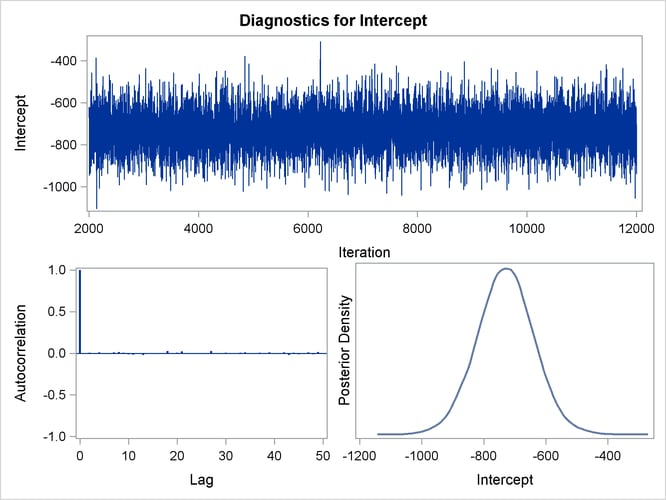Figure 40.21: Diagnostic Plots for logX1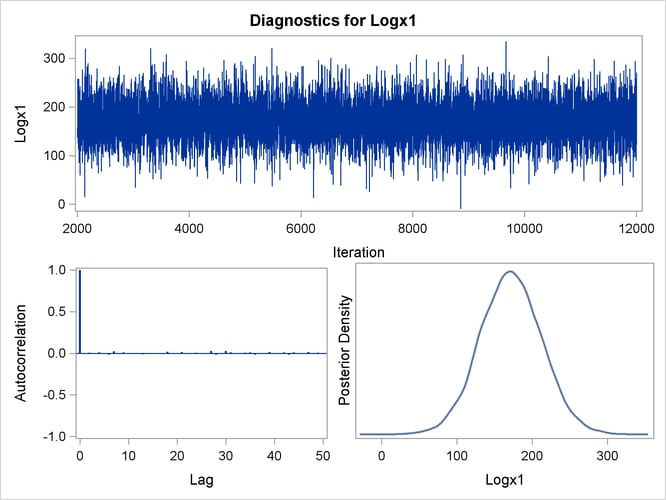Figure 40.22: Diagnostic Plots for X2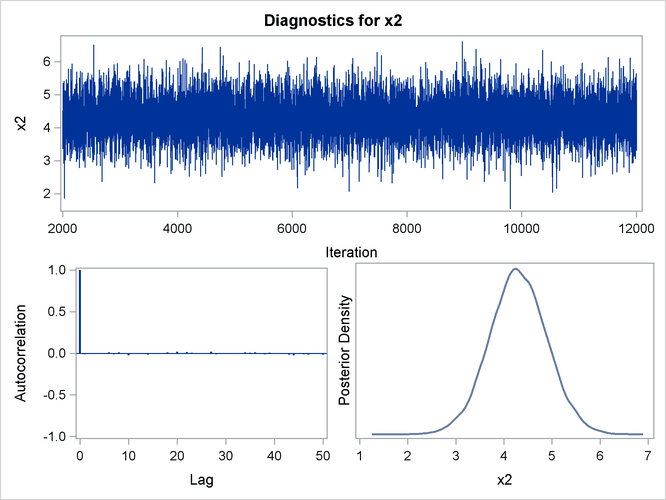Figure 40.23: Diagnostic Plots for X3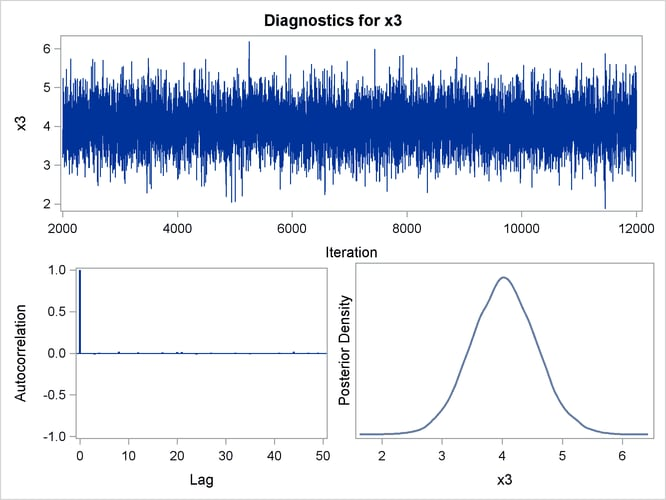Figure 40.24: Diagnostic Plots for X4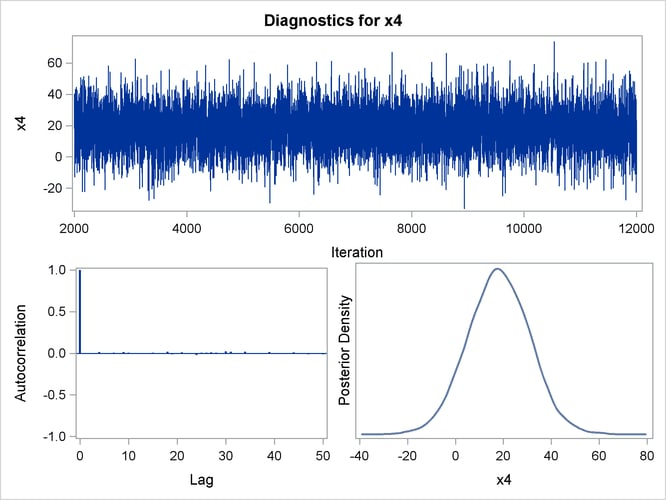Figure 40.25: Diagnostic Plots for X5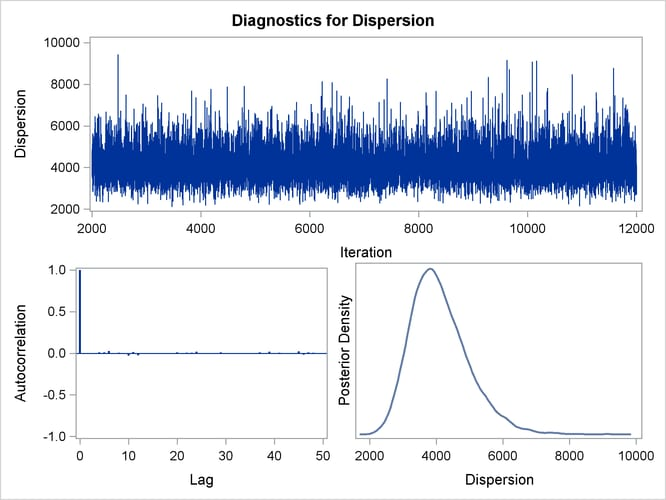Suppose, for illustration, a question of scientific interest is whether blood clotting score has a positive effect on survival time. Since the model parameters are regarded as random quantities in a Bayesian analysis, you can answer this question by estimating the conditional probability ofbeing positive, given the data,, from the posterior distribution samples. The following SAS statements compute the estimate of the probability ofbeing positive:

data Prob;
set PostSurg;
Indicator = (logX1 > 0);
label Indicator= 'log(Blood Clotting Score) > 0';
run;

proc Means data = Prob(keep=Indicator) n mean;
run;


As shown in Figure 40.26, there is a 1.00 probability of a positive relationship between the logarithm of a blood clotting score and survival time, adjusted for the other covariates.

Figure 40.26: Probability That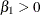The MEANS Procedure

Analysis Variable : Indicator
log(Blood Clotting Score)
> 0
N Mean
10000 0.9999000